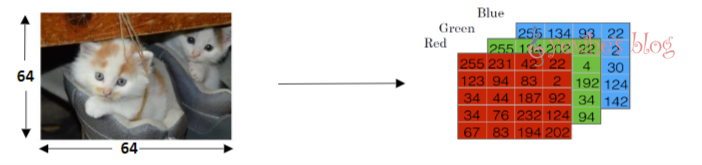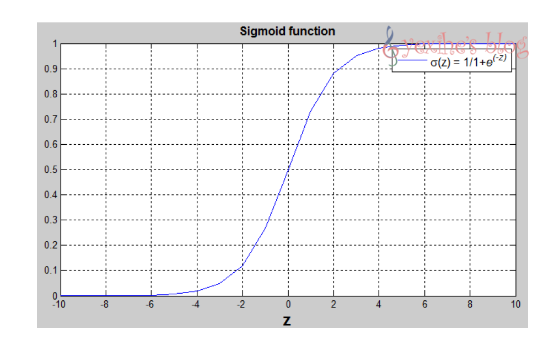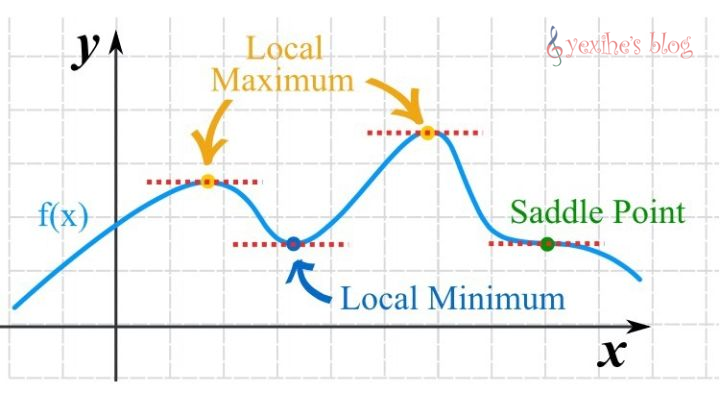# PyTorch入门

#### 为什么使用PyTorch

PyTorch 是 PyTorch 在 Python 上的衍生. 因为 PyTorch 是一个使用 PyTorch 语言的神经网络库, Torch 很好用, 但是 Lua 又不是特别流行, 所有开发团队将 Lua 的 Torch 移植到了更流行的语言 Python 上.• 与Tensorflow不同的是：
• Tensorflow先搭建静态流程图然后代入数据进行计算；
• PyTorch边代入数据边构建图；

#### 二分分类

In a binary classification problem,the result is a discrete value output.

##### Example：Cat vs Non-Cat#### Logistic回归

Logistic回归是一种用于有监督学习二分分类问题的学习算法。logistic回归的目标是使预测值与训练数据之间的误差最小化。

##### Example：Cat vs Non-Cat

Logistic回归中使用的参数有：

• 输入特征向量：$x \in R^nx$

• 训练特征标签：$y = 1|0$

• 权重：$w \in R^nx$

• 阈值：$b \in R$

• 输出: $y = \sigma(W^Tx+b)$

• Sigmoid函数：$s = \sigma(W^Tx+b)=\sigma(z)=1/(1+e^{-z})$#### logistic 回归函数#### 梯度下降法

##### 梯度下降与梯度上升

###### 梯度下降法#### 参考教程

-------------------本文结束 感谢您的阅读-------------------